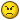# Drag Coefficient Of a Sphere In Hypersonic Flow

## Recommended Posts

Well, I'm supposed to compute the drag coefficient of a sphere in a hypersonic newtonian flow. In a newtonian flow the pressure coefficient is given by:

$C_p = sin(\alpha)$, with alpha the angle of attack.

The pressure coefficient on the lee side is 0.

Now. My problem is that I have to compute the total drag coefficient, integrating a surface integral over half a sphere. The final drag coefficient is supposed to be 1, but I just can't get it right, and my calculus book is a good 1000km away...Could someone help me out?

Thanx a lot!

##### Share on other sites

can you post what you've done so far? this would help us quite a bit.

##### Share on other sites

Well, I tried for a cylinder in a flow so far, and got the right answer. It's supposed to be a cylinder of length 1, and radius R.

$C_p = 2 sin^2(\alpha)$

$C_n=\frac{1}{c} \cdot \int_0^c C_p \: dr$

with c = 2R (the frontal surface of the cylinder, seen by the airflow)

$C_d = C_n \cdot sin(\alpha)$

$\Rightarrow C_d=\frac{1}{2R} \cdot \int_0^{\pi} 2sin^2(\alpha)\cdot sin(\alpha)\cdot R \cdot d\alpha$

Which, after integration by parts etc etc yields:

$C_d = \frac{4}{3}$

The point is: I'm not sure I did it the right way, I just got the right answer..For the sphere I started the same way, but with $c = \pi R^2$.

I put the theory in attachement for clarity, the example they use is for a flat plate.. Hope it will help..

Thanx again.

Newton.zip

Newton2.zip

## Create an account

Register a new account